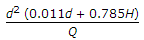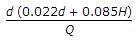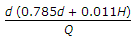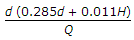# Online Civil Engineering Test - Waste Water Engineering TestLoading Test...

Instruction:

• This is a FREE online test. DO NOT pay money to anyone to attend this test.
• Total number of questions : 20.
• Time alloted : 30 minutes.
• Each question carry 1 mark, no negative marks.
• DO NOT refresh the page.
• All the best :-).

1.

The detention time of a circular tank of diameter d and water depth H, for receiving the sewage Q per hour, is

A.B.C.D.2.

In primary sedimentation, the 0.2 mm inorganic solids get separated if specific gravity is

A.
 2.25B.
 2.5C.
 2.55D.
 2.65E.
 2.753.

Disposal to sewage in large cities, is done in

A.
 irrigationB.
 dilutionC.
 oxidationD.
 putrifaction.4.

For evaporation and measurement of settlable solids, the apparatus used, is

A.
 a jarB.
 a breakerC.
 a test tubeD.
 an Imhoff cone.5.

In SI units the power of sound is represented in

A.
 kgsB.
 joulesC.
 neutonsD.
 watts.6.

Rate of flow of sewage is generally assumed

A.
 more than the rate of water supplyB.
 equal to the rate of water supplyC.
 less than the rate of water supplyD.
 at 150 litres per capita.7.

In case of Imhoff tanks,

A.
 the shape is rectangularB.
 detention period is 2 hoursC.
 the velocity of flow is restricted to 0.30 m/minuteD.
 surface loading is limited to 30, 000 litres/m of plan area per dayE.
 All the above.8.

In sewers the gas generally found, is

A.
 Hydrogen sulphide (H2S)B.
 Carbon dioxide (CO2)C.
 Methane (CH4)D.
 Petrol vapoursE.
 All the above.9.

Dry water flow in a combined sewer, is

A.
 industrial sewageB.
 domestic sewageC.
 storm waterD.
 inclusive of domestic and industrial sewage but excludes storm water.10.

Which one of the following tests is used for testing sewer pipes :

A.
 water testB.
 ball testC.
 mirror testD.
 all of these.11.

The self-cleansing velocity of water flowing through pipe lines, is

A.
 2 metres/secB.
 1 metre/secC.
 0.5 metre/secD.
 0.25 metre/sec.12.

Which one of the following part of human body withstands minimum radiation

A.
 thyroidB.
 kidneysC.
 eyesD.
 ovaries/testis.13.

Primary treatment of sewage consists of removal of

A.
 large suspended organic solidsB.
 oil and greaseC.
 sand and girtD.
 floating materialsE.
 none of these.14.

If D is the diameter of upper circular portion, the overall depth of New Egg shaped sewer section, is

A.
 1.250 DB.
 1.350 DC.
 1.425 DD.
 1.625 D.15.

In the activated sludge process

A.
 aeration is continued till stabilityB.
 aeration is done with an admixture of previously aerated sludgeC.
 sludge is activated by constant stirringD.
 water is removed by centrifugal action.16.

The sewerage system consists of

A.
 house sewerB.
 lateral sewerC.
 branch sewerD.
 main sewerE.
 all of these.17.

The laying of sewers is done with

A.
 magnetic compassB.
 theodoliteC.
 levelD.
 clinometerE.
 plane table.18.

In a trickling filter

A.
 filtration process is usedB.
 biological action is usedC.
 neither (a) nor (b)D.
 both (a) and (b).19.

In a grit chamber of a sewage treatment plant,

A.
 flow velocity 0.15 m to 0.3 m/sec is keptB.
 depth of 0.9 m to 1.20 m is keptC.
 one minute of detention period is keptD.
 all the above.20.

The most efficient cross-section of sewers in a combined sewerage system is

A.
 parabolicB.
 circularC.
 rectangularD.
 new egged.